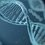# When $f^{-1}(x) = [f(x)]^{-1}$

Hello, members of the Brilliant community. Does anyone know how to find a function $f(x)$ if its inverse is equal to its reciprocal? I have been trying to come up with ways to do so, but to no avail.

$f^{-1}(x) = [f(x)]^{-1} = \frac{1}{f(x)}$Note by Ralph James
5 years, 2 months ago

This discussion board is a place to discuss our Daily Challenges and the math and science related to those challenges. Explanations are more than just a solution — they should explain the steps and thinking strategies that you used to obtain the solution. Comments should further the discussion of math and science.

When posting on Brilliant:

• Use the emojis to react to an explanation, whether you're congratulating a job well done , or just really confused .
• Ask specific questions about the challenge or the steps in somebody's explanation. Well-posed questions can add a lot to the discussion, but posting "I don't understand!" doesn't help anyone.
• Try to contribute something new to the discussion, whether it is an extension, generalization or other idea related to the challenge.
• Stay on topic — we're all here to learn more about math and science, not to hear about your favorite get-rich-quick scheme or current world events.

MarkdownAppears as
*italics* or _italics_ italics
**bold** or __bold__ bold
- bulleted- list
• bulleted
• list
1. numbered2. list
1. numbered
2. list
Note: you must add a full line of space before and after lists for them to show up correctly
paragraph 1paragraph 2

paragraph 1

paragraph 2

[example link](https://brilliant.org)example link
> This is a quote
This is a quote
    # I indented these lines
# 4 spaces, and now they show
# up as a code block.

print "hello world"
# I indented these lines
# 4 spaces, and now they show
# up as a code block.

print "hello world"
MathAppears as
Remember to wrap math in $$ ... $$ or $ ... $ to ensure proper formatting.
2 \times 3 $2 \times 3$
2^{34} $2^{34}$
a_{i-1} $a_{i-1}$
\frac{2}{3} $\frac{2}{3}$
\sqrt{2} $\sqrt{2}$
\sum_{i=1}^3 $\sum_{i=1}^3$
\sin \theta $\sin \theta$
\boxed{123} $\boxed{123}$

## Comments

Sort by:

Top Newest

I think the solution suggested by Oliver Daugherty-Long is correct.

If $f(x)=x^{i}$, where $i=\sqrt{-1}$,

then $f^{-1}(x)=x^{\frac{1}{i}}=x^{-i}=\frac{1}{x^i}=\frac{1}{f(x)}$

- 5 years, 2 months ago

Log in to reply

Okay, but are there any $f:\mathbb{R}\to \mathbb{R}$?

EDIT: Nope, again $f^{-1}$ doesn't exist. Take $x = e^{2\pi n}$.

- 5 years, 2 months ago

Log in to reply

That makes sense. $f^{-1}(x)$ would turn out be a multi-valued function in this case.

- 5 years, 2 months ago

Log in to reply

Does y=x^i work?

- 5 years, 2 months ago

Log in to reply

HInt: Suppose that $f(4) = 5$.

What can we say about $f(5) , f ( \frac{1}{4} ), f ( \frac{1}{5} )$?

Staff - 5 years, 2 months ago

Log in to reply

I take the function as $f(x)=x^i$. It satisfy the given condition

- 5 years, 2 months ago

Log in to reply

Then $f^{-1}$ will not be defined.

EDIT: Original function mentioned was $f(x) = 1$ New function still does not have an inverse defined.

- 5 years, 2 months ago

Log in to reply

OOps Sorry!

- 5 years, 2 months ago

Log in to reply

$f^{-1}(x) = \frac{1}{f(x)}$

Let $y = f^{-1}(x)$, i.e. $x= f(y)$.

$f^{-1}(f(y)) = \frac{1}{f(f(y))}$

$y = \frac{1}{f(f(y))}$

$f(f(y)) = \frac{1}{y}$

Maybe that helps...?

Edit 1: I just realised that the existence of an inverse proves that f(x) is bijective.

Therefore, if $f(x) = f(y)$, $x = y$, and vice versa.

$f(f(y)) = \frac{1}{y}$

$f(f(\frac{1}{y})) = y = f(f^{-1}(y))$

$f(\frac{1}{y}) =f^{-1}(y) = \frac{1}{f(y)}$

And we get that f(1) and f(-1) equals 1 or -1, f(1) not equal to f(-1).

i.e. $f(1)=1, f(-1)=-1$ or $f(1) = -1, f(-1) = 1$

- 5 years, 2 months ago

Log in to reply

Adding on, this gives, $f(f(f(f(y)))) = y$

- 5 years, 2 months ago

Log in to reply

In general Consider f(x) =y then replace all the x with y and y with x Then find y interms of x if u have one value for y,then that itself is inverse of f(x)

- 5 years, 2 months ago

Log in to reply

One I can think of is 1/x, but it wasn't defined for $x= 0$

- 5 years, 2 months ago

Log in to reply

$f^{-1}(x) = f(x)$ then, sadly not what we wanted.

- 5 years, 2 months ago

Log in to reply

×

Problem Loading...

Note Loading...

Set Loading...